0# Retain standard deviation values when removing a column

Hi there,

I currently have the following grid: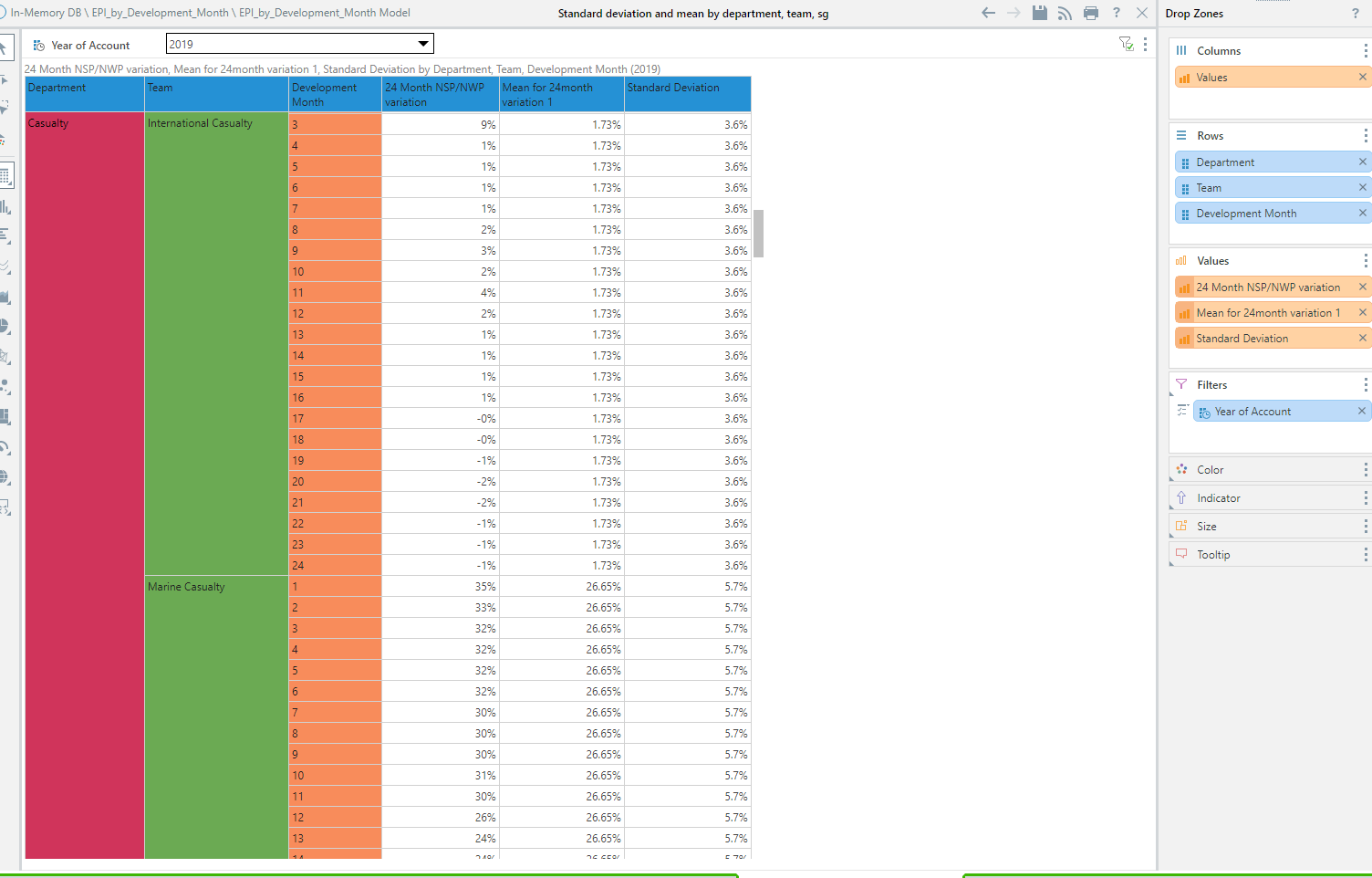I'd like to attain a grid that doesn't include the Development Month column but retains the standard deviation result (shown above) for each department and team.

If I remove the Development Month column, I get the following: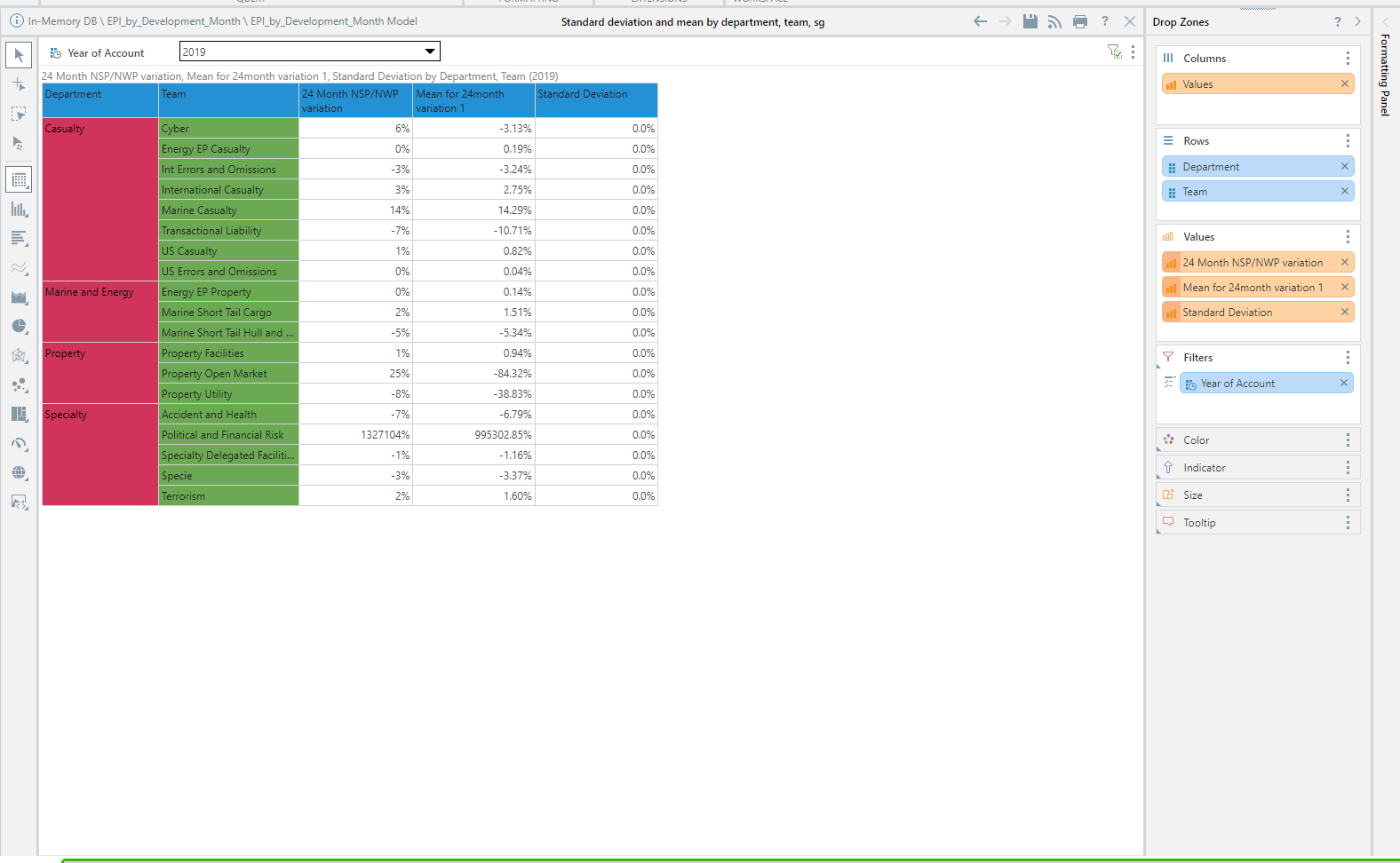Not only has the Standard Deviation value altered to 0, the other measures values have also changed from the previous screenshot. Though, the only measure/column I want to retain is the Standard Deviation one.

Essentially,  how can I get those standard deviation values from the first screenshot to appear in one row next to its corresponding team, as laid out in the second screenshot?

Below is the formulate I am using for context: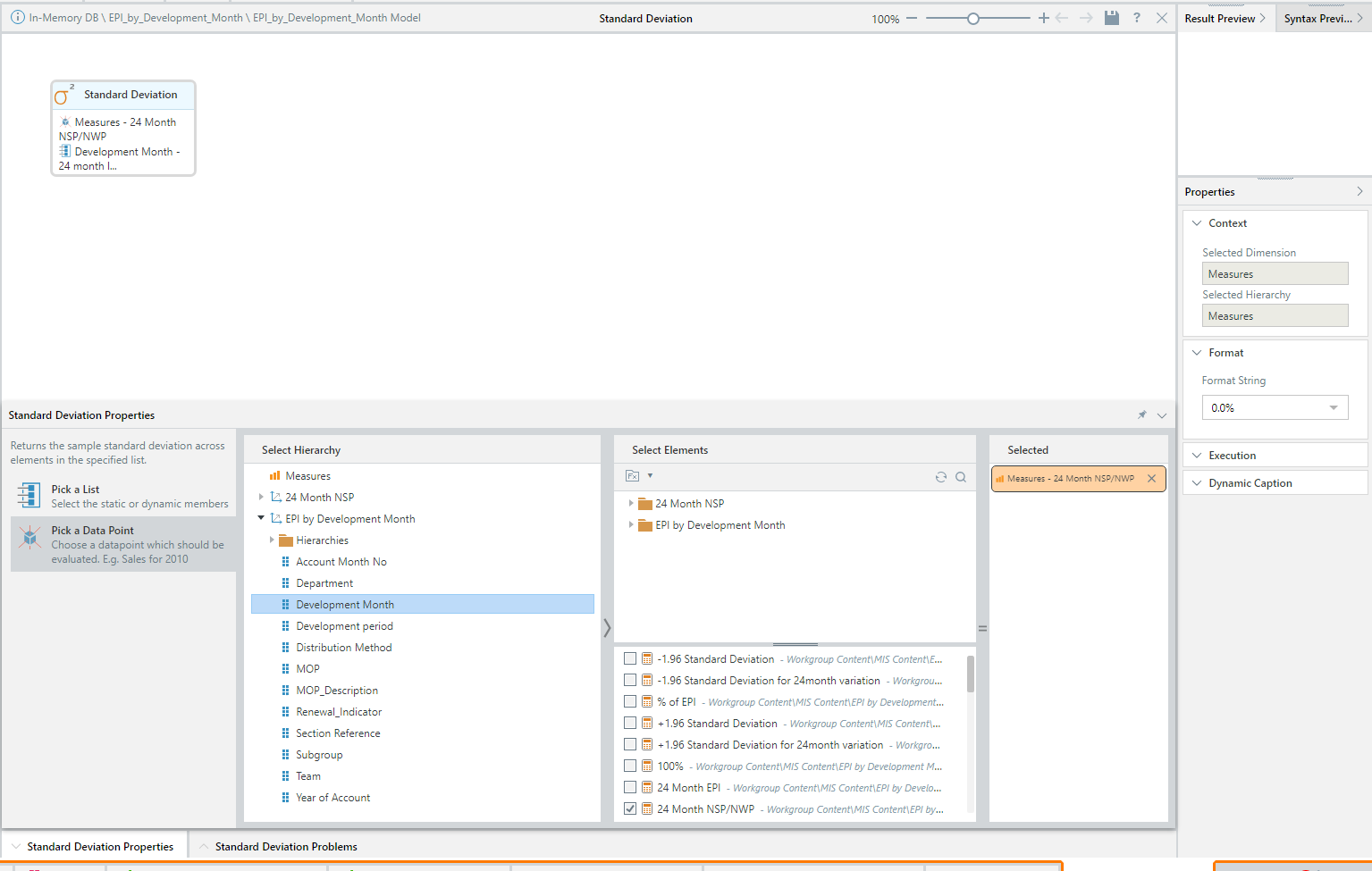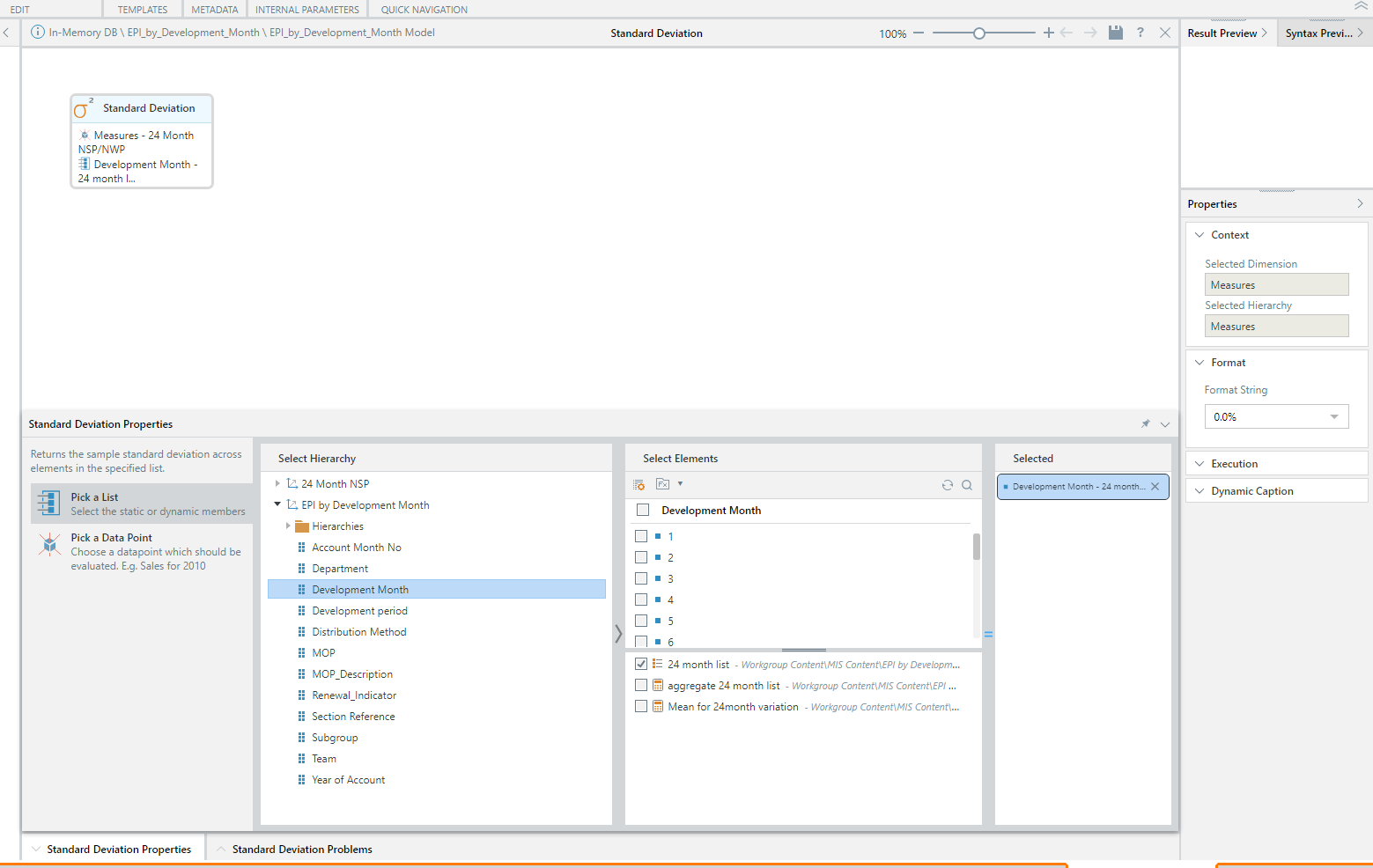Many thanks,

Ben

2replies Oldest first
• Oldest first
• Popular•To provide some additional context, the details on some of the formulas in use are as follows:

The 24 month NSP/NWP variation takes the value of the Net Signed Premium (denoted as NSP) at 24 months and divides it by the Net Written Premium (NWP Cumulative) for a given month. The purpose is to get an understanding of the deviation between these two values as, in a perfect world, they should equate to one another.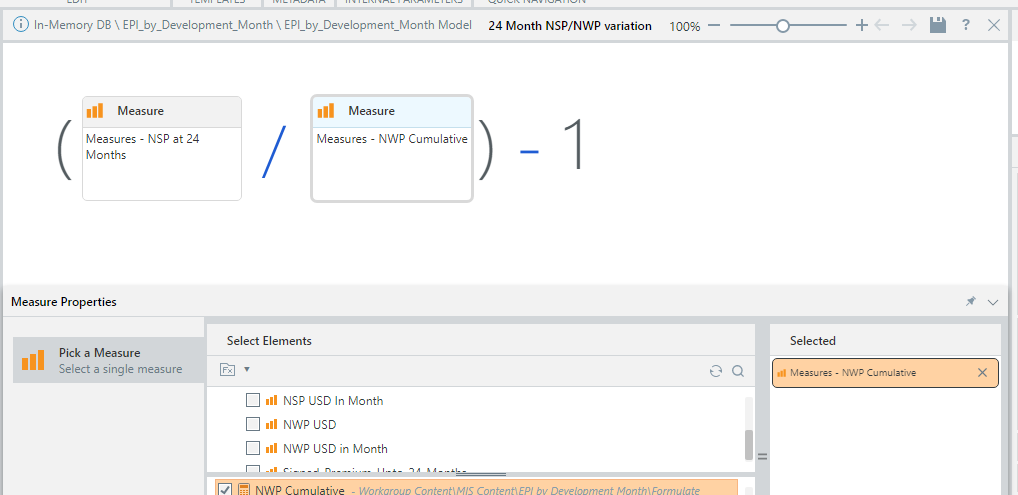The NWP Cumulative formula, which is being used above, is a bit more self-explanatory and is as follows: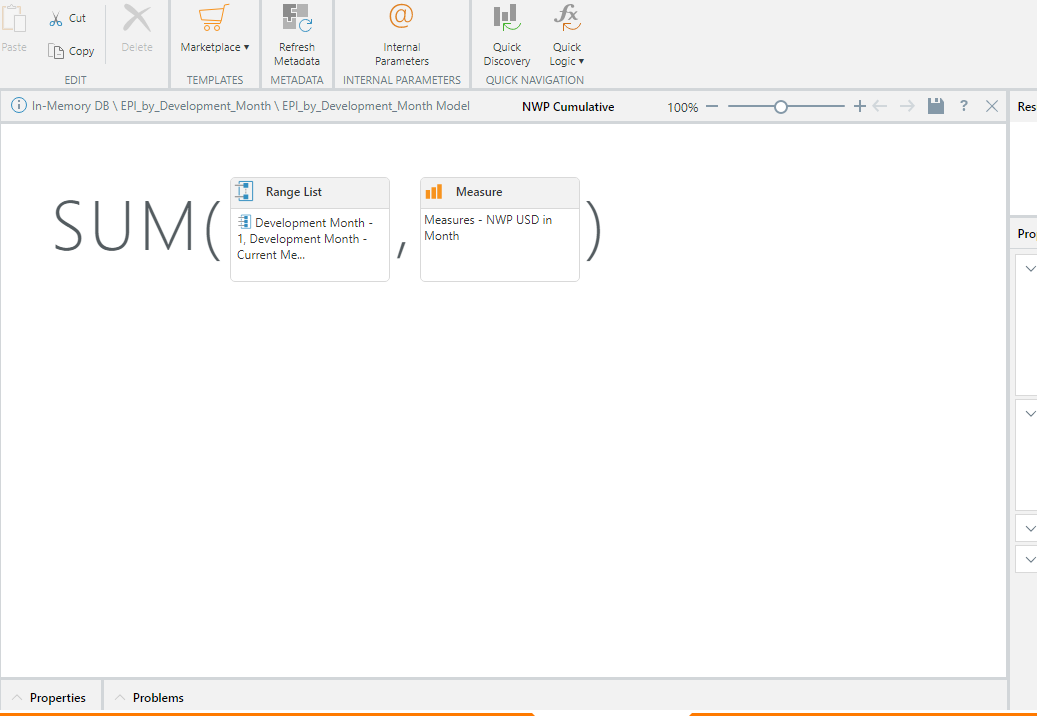The details behind the members being selected in the 24 month list (as part of the Standard Deviation formula) are as follows:

1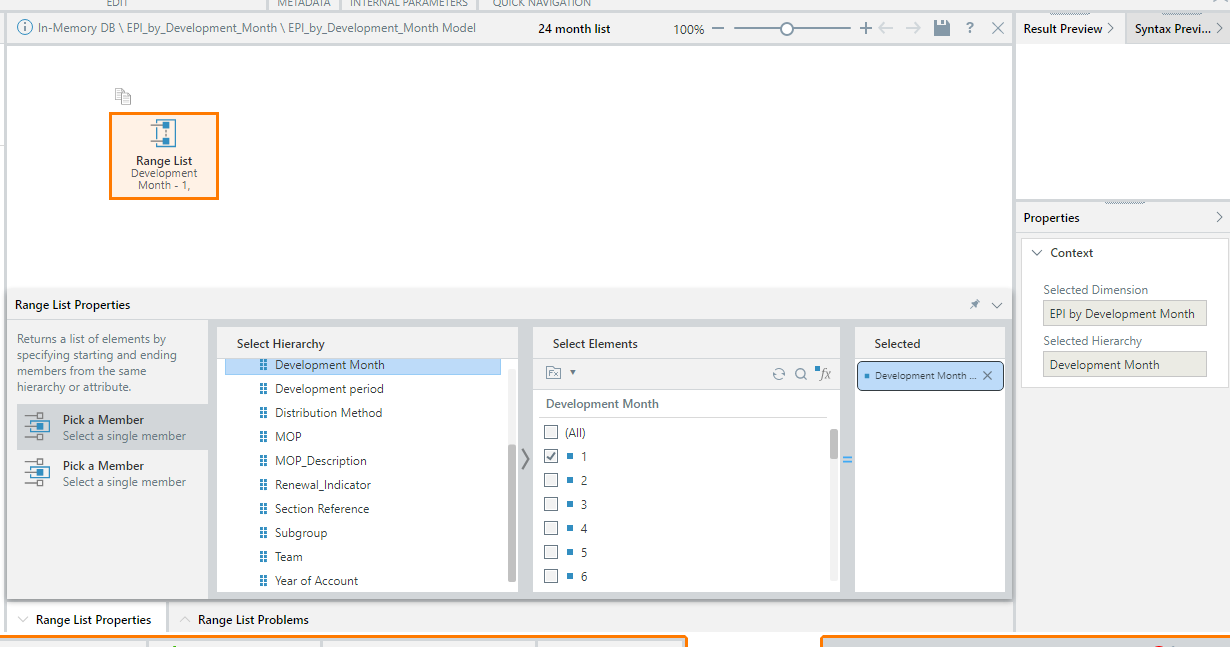to 24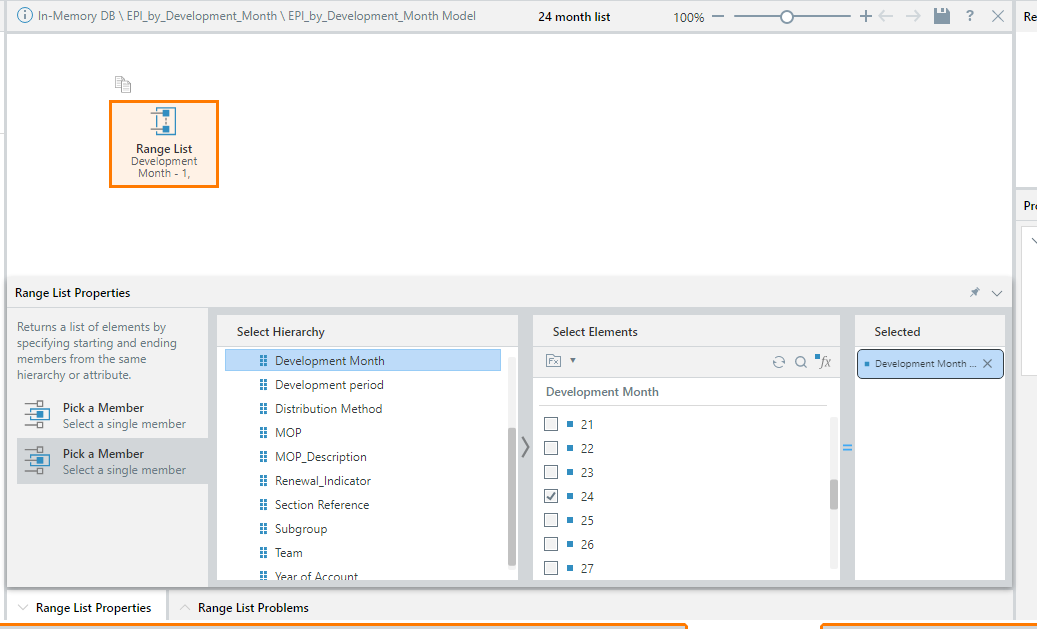If there are any further details I can provide, then do not hesitate to ask me.

Thanks,

Ben

Like
•Hi Benjamin, If the cumulative NWP looks at the current member for month and there is no current Month member in the query (when you remove the chip from the drop zone), then the query will not be able to return a value. It would be best to use a range list for the cumulative measure that does not depend on a current member (like 1 to 24). The derived value should then resolve correctly.

Like
Like Follow